$\newcommand{\dotp}{\langle #1, #2 \rangle}$ $\newcommand{\enscond}{\lbrace #1, #2 \rbrace}$ $\newcommand{\pd}{ \frac{ \partial #1}{\partial #2} }$ $\newcommand{\umin}{\underset{#1}{\min}\;}$ $\newcommand{\umax}{\underset{#1}{\max}\;}$ $\newcommand{\umin}{\underset{#1}{\min}\;}$ $\newcommand{\uargmin}{\underset{#1}{argmin}\;}$ $\newcommand{\norm}{\|#1\|}$ $\newcommand{\abs}{\left|#1\right|}$ $\newcommand{\choice}{ \left\{ \begin{array}{l} #1 \end{array} \right. }$ $\newcommand{\pa}{\left(#1\right)}$ $\newcommand{\diag}{{diag}\left( #1 \right)}$ $\newcommand{\qandq}{\quad\text{and}\quad}$ $\newcommand{\qwhereq}{\quad\text{where}\quad}$ $\newcommand{\qifq}{ \quad \text{if} \quad }$ $\newcommand{\qarrq}{ \quad \Longrightarrow \quad }$ $\newcommand{\ZZ}{\mathbb{Z}}$ $\newcommand{\CC}{\mathbb{C}}$ $\newcommand{\RR}{\mathbb{R}}$ $\newcommand{\EE}{\mathbb{E}}$ $\newcommand{\Zz}{\mathcal{Z}}$ $\newcommand{\Ww}{\mathcal{W}}$ $\newcommand{\Vv}{\mathcal{V}}$ $\newcommand{\Nn}{\mathcal{N}}$ $\newcommand{\NN}{\mathcal{N}}$ $\newcommand{\Hh}{\mathcal{H}}$ $\newcommand{\Bb}{\mathcal{B}}$ $\newcommand{\Ee}{\mathcal{E}}$ $\newcommand{\Cc}{\mathcal{C}}$ $\newcommand{\Gg}{\mathcal{G}}$ $\newcommand{\Ss}{\mathcal{S}}$ $\newcommand{\Pp}{\mathcal{P}}$ $\newcommand{\Ff}{\mathcal{F}}$ $\newcommand{\Xx}{\mathcal{X}}$ $\newcommand{\Mm}{\mathcal{M}}$ $\newcommand{\Ii}{\mathcal{I}}$ $\newcommand{\Dd}{\mathcal{D}}$ $\newcommand{\Ll}{\mathcal{L}}$ $\newcommand{\Tt}{\mathcal{T}}$ $\newcommand{\si}{\sigma}$ $\newcommand{\al}{\alpha}$ $\newcommand{\la}{\lambda}$ $\newcommand{\ga}{\gamma}$ $\newcommand{\Ga}{\Gamma}$ $\newcommand{\La}{\Lambda}$ $\newcommand{\si}{\sigma}$ $\newcommand{\Si}{\Sigma}$ $\newcommand{\be}{\beta}$ $\newcommand{\de}{\delta}$ $\newcommand{\De}{\Delta}$ $\newcommand{\phi}{\varphi}$ $\newcommand{\th}{\theta}$ $\newcommand{\om}{\omega}$ $\newcommand{\Om}{\Omega}$ $\newcommand{\eqdef}{\equiv}$

This numerical tour explore several extensions of the basic Sinkhorn method.

In :
import numpy as np
import matplotlib.pyplot as plt


Log-domain Sinkhorn ¶

For simplicity, we consider uniform distributions on point clouds, so that the associated histograms are $(a,b) \in \RR^n \times \RR^m$ being constant $1/n$ and $1/m$.

In :
n = 100
m = 200
a = np.ones((n,1))/n
b = np.ones((1,m))/m


Point clouds $x$ and $y$.

In :
x = np.random.rand(2,n)-.5
theta = 2*np.pi*np.random.rand(1,m)
r = .8 + .2*np.random.rand(1,m)
y = np.vstack((np.cos(theta)*r,np.sin(theta)*r))


Display of the two clouds.

In :
plotp = lambda x,col: plt.scatter(x[0,:], x[1,:], s=150, edgecolors="k", c=col, linewidths=2)
plt.figure(figsize=(6,6))
plotp(x, 'b')
plotp(y, 'r')
plt.axis("off");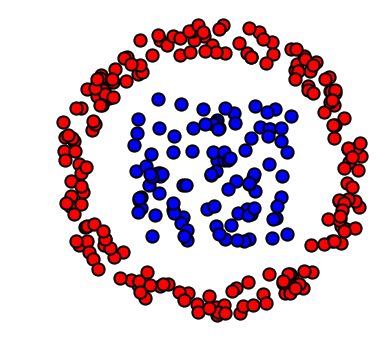Cost matrix $C_{i,j} = \norm{x_i-y_j}^2$.

In :
def distmat(x,y):
return np.sum(x**2,0)[:,None] + np.sum(y**2,0)[None,:] - 2*x.transpose().dot(y)
C = distmat(x,y)


Sinkhorn algorithm is originally implemented using matrix-vector multipliciation, which is unstable for small epsilon. Here we consider a log-domain implementation, which operates by iteratively updating so-called Kantorovitch dual potentials $(f,g) \in \RR^n \times \RR^m$.

The update are obtained by regularized c-transform, which reads $$f_i \leftarrow {\min}_\epsilon^b( C_{i,\cdot} - g )$$ $$g_j \leftarrow {\min}_\epsilon^a( C_{\cdot,j} - f ),$$ where the regularized minimum operator reads $${\min}_\epsilon^a(h) \eqdef -\epsilon \log \sum_i a_i e^{-h_i/\epsilon}.$$

In :
def mina_u(H,epsilon): return -epsilon*np.log( np.sum(a * np.exp(-H/epsilon),0) )
def minb_u(H,epsilon): return -epsilon*np.log( np.sum(b * np.exp(-H/epsilon),1) )


The regularized min operator defined this way is non-stable, but it can be stabilized using the celebrated log-sum-exp trick, wich relies on the fact that for any constant $c \in \RR$, one has $${\min}_\epsilon^a(h+c) = {\min}_\epsilon^a(h) + c,$$ and stabilization is achieved using $c=\min(h)$.

In :
def mina(H,epsilon): return mina_u(H-np.min(H,0),epsilon) + np.min(H,0);
def minb(H,epsilon): return minb_u(H-np.min(H,1)[:,None],epsilon) + np.min(H,1);


Value of $\epsilon$.

In :
epsilon = .01


Exercise 1

Implement Sinkhorn in log domain.

In :
def Sinkhorn(C,epsilon,f,niter = 500):
Err = np.zeros(niter)
for it in range(niter):
g = mina(C-f[:,None],epsilon)
f = minb(C-g[None,:],epsilon)
# generate the coupling
P = a * np.exp((f[:,None]+g[None,:]-C)/epsilon) * b
# check conservation of mass
Err[it] = np.linalg.norm(np.sum(P,0)-b,1)
return (P,Err)
# run with 0 initialization for the potential f
(P,Err) =  Sinkhorn(C,epsilon,np.zeros(n),3000)
plt.plot(np.log10(Err));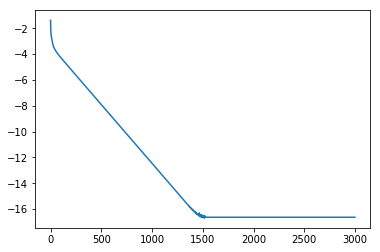Exercise 2

Study the impact of $\epsilon$ on the convergence rate of the algorithm.

In :
for epsilon in (.1, .05, .01, .001):
(P,Err) =  Sinkhorn(C,epsilon,np.zeros(n),1000)
plt.plot(np.log10(Err), label='$\epsilon=$' + str(epsilon))
plt.legend();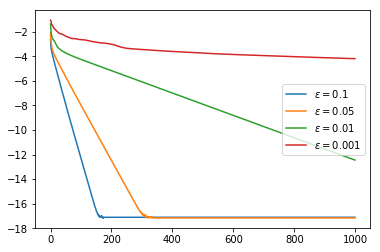Wasserstein Flow for Matching ¶

We aim at performing a "Lagrangian" gradient (also called Wasserstein flow) descent of Wasserstein distance, in order to perform a non-parametric fitting. This corresponds to minimizing the energy function $$\Ee(z) \eqdef W_\epsilon\pa{ \frac{1}{n}\sum_i \de_{z_i}, \frac{1}{m}\sum_i \de_{y_i} }.$$

Here we have denoted the Sinkhorn score as $$W_\epsilon(\al,\be) \eqdef \dotp{P}{C} - \epsilon \text{KL}(P|ab^\top)$$ where $\al=\frac{1}{n}\sum_i \de_{x_i}$ and $\be=\frac{1}{m}\sum_i \de_{y_i}$ are the measures (beware that $C$ depends on the points positions).

In :
z = x # initialization


The gradient of this energy reads $$( \nabla \Ee(z) )_i = \sum_j P_{i,j}(z_i-y_j) = a_i z_i - \sum_j P_{i,j} y_j,$$ where $P$ is the optimal coupling. It is better to consider a renormalized gradient, which corresponds to using the inner product associated to the measure $a$ on the deformation field, in which case $$( \bar\nabla \Ee(z) )_i = z_i - \bar y_i \qwhereq \bar y_i \eqdef \frac{\sum_j P_{i,j} y_j}{a_i}.$$ Here $\bar y_i$ is often called the "barycentric projection" associated to the coupling matrix $P$.

First run Sinkhorn, beware you need to recompute the cost matrix at each step.

In :
epsilon = .01
niter = 300
(P,Err) = Sinkhorn(distmat(z,y), epsilon,np.zeros(n),niter);


In :
G = z - ( y.dot(P.transpose()) ) / a.transpose()


In :
plt.figure(figsize=(6,6))
plotp(x, 'b')
plotp(y, 'r')
for i in range(n):
plt.plot([x[0,i], x[0,i]-G[0,i]], [x[1,i], x[1,i]-G[1,i]], 'k')
plt.axis("off");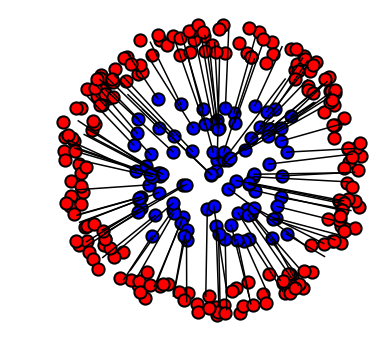Set the descent step size.

In :
tau = .1


Update the point cloud.

In :
z = z - tau * G


Exercise 3

In :
z = x; # initialization
tau = .03; # step size for the descent
giter = 20; # iter for the gradient descent
ndisp = np.round( np.linspace(0,giter-1,6) )
kdisp = 0
f = np.zeros(n) # use warm restart in the following
for j in range(giter):
# drawing
if ndisp[kdisp]==j:
plt.subplot(2,3,kdisp+1)
s = j/(giter-1)
col = np.array([s,0,1-s])[None,:]
plotp(z, col )
plt.axis("off")
kdisp = kdisp+1
# Sinkhorn
(P,Err) = Sinkhorn(distmat(z,y), epsilon,f,niter)
G = z - ( y.dot(P.transpose()) ) / a.transpose()
z = z - tau * G;Exercise 4

Show the evolution of the fit as $\epsilon$ increases. What do you observe. Replace the Sinkhorn score $W_\epsilon(\al,\be)$ by the Sinkhorn divergence $W_\epsilon(\al,\be)-W_\epsilon(\al,\al)/2-W_\epsilon(\be,\be)/2$.

In :
## Insert your code here.


Generative Model Fitting¶

The Wasserstein is a non-parametric idealization which does not corresponds to any practical application. We consider here a simple toy example of density fitting, where the goal is to find a parameter $\theta$ to fit a deformed point cloud of the form $(g_\theta(x_i))_i$ using a Sinkhorn cost. This is ofen called a generative model in the machine learning litterature, and corresponds to the problem of shape registration in imaging.

The matching is achieved by solving $$\min_\th \Ff(\th) \eqdef \Ee(G_\th(z)) = W_\epsilon\pa{ \frac{1}{n}\sum_i \de_{g_\th(z_i)}, \frac{1}{m}\sum_i \de_{y_i} },$$ where the function $G_\th(z)=( g_\th(z_i) )_i$ operates independently on each point.

The gradient reads $$\nabla \Ff(\th) = \sum_i \partial g_\th(z_i)^*[ \nabla \Ee(G_\th(z))_i ],$$ where $\partial g_\th(z_i)^*$ is the adjoint of the Jacobian of $g_\th$.

We consider here a simple model of affine transformation, where $\th=(A,h) \in \RR^{d \times d} \times \RR^d$ and $g_\th(z_i)=Az_i+h$.

Denoting $v_i = \nabla \Ee(G_\th(z))_i$ the gradient of the Sinkhorn loss (which is computed as in the previous section), the gradient with respect to the parameter reads $$\nabla_A \Ff(\th) = \sum_i v_i z_i^\top \qandq \nabla_h \Ff(\th) = \sum_i v_i.$$

Generate the data.

In :
z = np.random.randn(2,n)*.2
y = np.random.randn(2,m)*.2
y[0,:] = y[0,:]*.05 + 1


Initialize the parameters.

In :
A = np.eye(2)
h = np.zeros(2)


Display the clouds.

In :
plotp(A.dot(z)+h[:,None], 'b')
plotp(y, 'r')
plt.xlim(-.7,1.1)
plt.ylim(-.7,.7);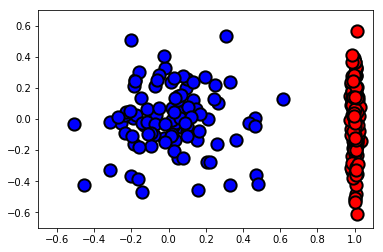Run Sinkhorn.

In :
x = A.dot(z)+h[:,None]
f = np.zeros(n)
(P,Err) = Sinkhorn(distmat(x,y), epsilon,f,niter)


Compute gradient with respect to positions.

In :
v = a.transpose() * x - y.dot(P.transpose())


In :
nabla_A = v.dot(z.transpose())
nabla_h = np.sum(v,1)


Exercise 5

In :
A = np.eye(2)
h = np.zeros(2)
# step size for the descent
tau_A = .8
tau_h = .1
# #iter for the gradient descent
giter = 40
ndisp = np.round( np.linspace(0,giter-1,6) )
kdisp = 0
f = np.zeros(n)
for j in range(giter):
x = A.dot(z)+h[:,None]
if ndisp[kdisp]==j:
plt.subplot(2,3,kdisp+1)
plotp(y, 'r')
plotp(x, 'b')
kdisp = kdisp+1
plt.xlim(-.7,1.3)
plt.ylim(-.7,.7)
(P,Err) = Sinkhorn(distmat(x,y), epsilon,f,niter)
v = a.transpose() * x - y.dot(P.transpose())
nabla_A = v.dot(z.transpose())
nabla_h = np.sum(v,1)
A = A - tau_A * nabla_A
h = h - tau_h * nabla_h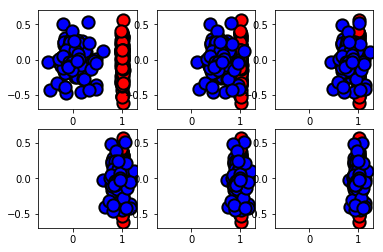Exercise 5

Test using a more complicated deformation (for instance a square being deformed by a random $A$.

In [ ]: